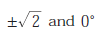# EC GATE 2009 (Test 3)

Tag: ec gate 2009
Q.1
A communication channel with AWGN operating at a signal to noise ration SNR >> 1 and bandwidth B has capacity C1. If the SNR is doubled keeping constant, the resulting capacity C2 is given by
A.B.C.D.Explaination / Solution:Workspace
Report
Q.2
A transmission line terminates in two branches, each of lengthas shown. The branches are terminated by 50W loads. The lines are lossless and have the characteristic impedances shown. Determine the impedance Zi as seen by the source.A. 200

B. 100

C. 50

D. 25

Explaination / Solution:

The transmission line are as shown below. Length of all line isWorkspace
Report
Q.3
The amplitude of a random signal is uniformly distributed between -5 V and 5 V.If the signal to quantization noise ratio required in uniformly quantizing the signal is 43.5 dB, the step of the quantization is approximately
A. 0.033 V
B. 0.05 V
C. 0.0667 V
D. 0.10 V
Explaination / Solution:Workspace
Report
Q.4
The magnitude plot of a rational transfer function G(s) with real coefficients is shown below. Which of the following compensators has such a magnitude plot ?B. Lag compensator
C. PID compensator
Explaination / Solution:

This compensator is roughly equivalent to combining lead and lad compensators in the same design and it is referred also as PID compensator.

Workspace
Report
Q.5
The feedback configuration and the pole-zero locations ofare shown below. The root locus for negative values of k , i.e. for -3 < k < 0, has breakaway/break-in points and angle of departure at pole P (with respect to the
positive real axis) equal toA.B.C.D.Explaination / Solution:

The characteristic equation isWorkspace
Report
Q.6
An LTI system having transfer functionand input x(t) = sin(t + 1) is in steady state. The output is sampled at a rate ws rad/s to obtain the final output {x(k)}. Which of the following is true ?
A. y(.) is zero for all sampling frequencies
ws
B. y(.) is nonzero for all sampling frequencies ws
C. y(.) is nonzero for ws > 2 , but zero for ws < 2
D. y(.) is zero for ws > 2, but nonzero for w2< 2
Explaination / Solution:
No Explaination.

Workspace
Report
Q.7
The unit step response of an under-damped second order system has steady state value of -2. Which one of the following transfer functions has theses properties ?
A.B.C.D.Explaination / Solution:

For under-damped second order responseWorkspace
Report
Q.8
The Nyquist plot of a stable transfer function G(s) is shown in the figure are interested in the stability of the closed loop system in the feedback configuration shown.Which of the following statements is true ?
A. G(s) is an all-pass filter
B. G(s) has a zero in the right-half plane
C. G(s) is the impedance of a passive network
D. G(s) is marginally stable
Explaination / Solution:

The plot has one encirclement of origin in clockwise direction. Thus G(s) has a zero is in RHP.

Workspace
Report
Q.9
The Nyquist plot of a stable transfer function G(s) is shown in the figure are interested in the stability of the closed loop system in the feedback configuration shown.The gain and phase margins of G(s) for closed loop stability are
A. 6 dB and 180◦C
B. 3 dB and 180◦C
C. 6 dB and 90 dB an
D. 3 dB and 90
Explaination / Solution:

The Nyzuist plot intersect the real axis ate - 0.5. Thus
G. M. =- 20 log x =- 20 log 0.5 = 6.020 dB
And its phase margin is 90c

Workspace
Report
Q.10
The Fourier series of a real periodic function has only (P) cosine terms if it is even (Q) sine terms if it is even (R) cosine terms if it is odd (S) sine terms if it is odd Which of the above statements are correct ?
A. P and S
B. P and R
C. Q and S
D. Q and R